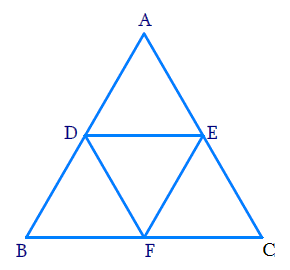# Ex.6.4 Q5 Triangles Solution - NCERT Maths Class 10

Go back to  'Ex.6.4'

## Question

$$D, E$$ and $$F$$ are respectively the mid-points of sides $$AB, BC$$ and $$CA$$ of $$\Delta \,ABC.$$ Find the ratio of the areas of $$\Delta \,DEF$$ and $$\Delta \,ABC.$$

DiagramVideo Solution
Triangles
Ex 6.4 | Question 5

## Text Solution

Reasoning:

As we know that the line segment joining the midpoints of two sides of a triangle is parallel to the third side and half of it – (mid-point theorem).

Steps:

In $$\Delta ABC$$, $$D\, {\rm{}and}\, F$$ are the midpoints of $$AB, AC$$ respectively.

\begin{align} \Rightarrow \;\;&DE\parallel BC \;\text{ and }\; DE = \frac{1}{2}BC \\ &\text{(by mid-point theorem)} \end{align}

Again, $$E$$ is mid-point of $$BC$$

\begin{align} \Rightarrow \;\;DF \parallel BE \;\text{ and }\; DF = BE\end{align}

In quadrilateral $$DFEB,$$

\begin{align} DF \parallel BE \;\text{ and }\; DF = BE\end{align}

$$\therefore$$ $$DFEB$$ is a parallelogram

\begin{align}\Rightarrow \quad &\angle B = \angle F\;\;\dots(1) \\ &\begin{bmatrix} \text{Opposite angles of a } \\ \text{parallelogram are equal}\end{bmatrix} \end{align}

Similarly, we can prove that

$$DFCE$$ is a parallelogram

\begin{align}\Rightarrow \quad& \angle C=\angle D\;\;\dots(2)\\ &\begin{bmatrix} \text{Opposite angles of a } \\ \text{parallelogram are equal}\end{bmatrix} \end{align}

Now,

In $$\Delta DEF$$ and $$\Delta ABC$$

$\angle DEF = \angle ABC \;\;\dots \text{from (1)}$

$\angle EDF = \angle ACB \;\;\dots \text{from (2)}$

\begin{align}\Rightarrow \quad & \Delta DEF \sim \Delta CAB \\ & \text{ [AA Criterion]} \end{align}

The ratio of the areas of two similar triangles is equal to the square of corresponding sides.

\begin{align}\frac{{ \text{ar}\,\Delta DEF}}{{\text{ar}\,\Delta ABC}} & = \frac{{{{(DE)}^2}}}{{{{(AC)}^2}}} \\ & = \frac{{{{(EF)}^2}}}{{{{(AB)}^2}}} \\ & = \frac{{{{(DF)}^2}}}{{{{(BC)}^2}}}\end{align}

\begin{align} \frac{\text {ar} \Delta D E F}{\text {ar} \Delta A B C} &=\frac{(D F)^{2}}{(BC)^{2}} \\ &=\frac{(\frac12 BC)^{2}}{(BC)^{2}} \quad\\ &=\frac{B C^{2}}{4 B C^{2}}\\ &=\frac{{1}}{4} \end{align}

The ratio of the areas of $$\Delta DEF$$ and $$\Delta ABC$$ is $$1:4$$

Alternate  method:

Reasoning:

Mid-Point Theorem : The line segment joining the mid-points of two sides of a triangle is parallel to the third side.

Steps:

In $$\Delta ABC$$ ,$$D$$ and $$E$$ are midpoints of sides $$AB$$ and $$AC$$

$\Rightarrow DE\parallel BC$

and

\begin{align} DE \!=\! \frac{1}{2}BC \end{align} \dots (1)

Now in quadrilateral $$DBFE$$

$\Rightarrow DE\parallel BC \text{ and }DE \!=\! BF \dots\text{from (1)}$

$$\Rightarrow$$ $$DBFE$$ is a parallelogram

\begin{align} &\Rightarrow \quad ar\Delta DBF \!=\! ar \Delta DEF \;\;\dots(2) \\ &\begin{bmatrix}\because \text{Diagonal DF divides the parallelogram } \\ \text{into two triangles of equal area} \end{bmatrix}\end{align}

Similarly, we can prove

$ar\Delta DBF \! = \! ar\Delta EFC\;\; \dots (3)$

$ar\Delta DEF \! = \! ar\Delta ADE\;\; \dots (4)$

From $$(2 )$$ $$( 3)$$ and $$(4)$$

$\begin{bmatrix}ar\Delta DBF \! = \! ar\Delta DEF \! =\\ \! ar\Delta EFC \! = \! ar\Delta ADE\end{bmatrix}\;\;\dots(5)$

Things which are equal to the same thing are equal to one another $$–$$ Euclid’s $$1$$st axiom.

\begin{align} \begin{bmatrix} ar\Delta ABC = ar\Delta ADE = ar\Delta DBF = \\ar\Delta EFD = ar\Delta DEF \end{bmatrix} \end{align}

From $$(5)$$

\begin{align} ar\Delta ABC & \! = \! 4 \! \times \! ar\Delta DEF \\\frac{ar\Delta DEF}{ar\Delta ABC} & \! = \! \frac{1}{4}\\ar\Delta DEF : ar\Delta ABC & \! = \! 1 \! : \! 4 \end{align}

Learn from the best math teachers and top your exams

• Live one on one classroom and doubt clearing
• Practice worksheets in and after class for conceptual clarity
• Personalized curriculum to keep up with school